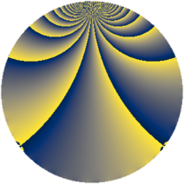# Properties

 Label 1148.2.pLevel $1148$ Weight $2$ Character orbit 1148.p Rep. character $\chi_{1148}(411,\cdot)$ Character field $\Q(\zeta_{6})$ Dimension $320$ Sturm bound $336$

# Learn more about

## Defining parameters

 Level: $$N$$ $$=$$ $$1148 = 2^{2} \cdot 7 \cdot 41$$ Weight: $$k$$ $$=$$ $$2$$ Character orbit: $$[\chi]$$ $$=$$ 1148.p (of order $$6$$ and degree $$2$$) Character conductor: $$\operatorname{cond}(\chi)$$ $$=$$ $$28$$ Character field: $$\Q(\zeta_{6})$$ Sturm bound: $$336$$

## Dimensions

The following table gives the dimensions of various subspaces of $$M_{2}(1148, [\chi])$$.

Total New Old
Modular forms 344 320 24
Cusp forms 328 320 8
Eisenstein series 16 0 16

## Trace form

 $$320q + 2q^{2} - 2q^{4} - 4q^{8} - 160q^{9} + O(q^{10})$$ $$320q + 2q^{2} - 2q^{4} - 4q^{8} - 160q^{9} - 30q^{12} - 6q^{14} - 18q^{16} - 16q^{21} + 16q^{22} + 152q^{25} + 32q^{28} - 32q^{29} + 16q^{30} - 8q^{32} - 24q^{33} + 20q^{36} + 8q^{37} - 54q^{42} - 26q^{44} + 4q^{46} - 16q^{49} - 8q^{50} + 54q^{52} - 8q^{53} - 30q^{54} + 2q^{56} + 80q^{57} - 4q^{58} - 24q^{60} + 24q^{61} - 20q^{64} - 16q^{65} + 30q^{68} + 66q^{70} + 18q^{72} - 72q^{73} - 2q^{74} - 16q^{77} - 96q^{78} + 60q^{80} - 144q^{81} + 44q^{84} - 48q^{85} + 44q^{86} + 4q^{88} - 64q^{92} - 8q^{93} - 102q^{96} + 60q^{98} + O(q^{100})$$

## Decomposition of $$S_{2}^{\mathrm{new}}(1148, [\chi])$$ into newform subspaces

The newforms in this space have not yet been added to the LMFDB.

## Decomposition of $$S_{2}^{\mathrm{old}}(1148, [\chi])$$ into lower level spaces

$$S_{2}^{\mathrm{old}}(1148, [\chi]) \cong$$ $$S_{2}^{\mathrm{new}}(28, [\chi])$$$$^{\oplus 2}$$